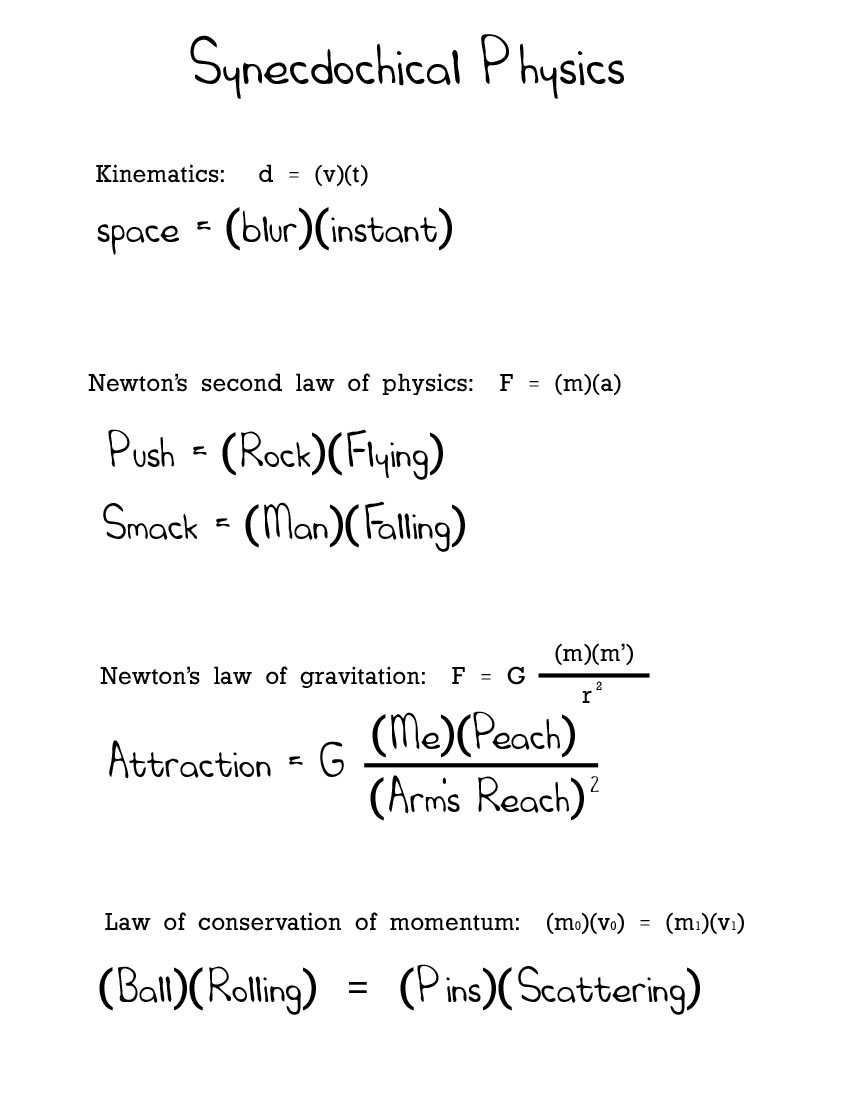# Synecdochical Physics

Kinematics:

d = vt ……………………………Space = (Blur)(Instant)

Newton's Second Law of Physics:

F = ma …………………………..Push = (Rock)(Flying)
F = ma …………………………..Smack = (Man)(Falling)

Newton's Law of Gravitation:

F = G(m)(m')/r^2 ……………..Attraction = G(Me)(Peach)/(Arm's Reach)^2

Law of conservation of momentum:

m1v1 = m2v2 ……………………(Ball)(Rolling) = (Pins)(Scattering)